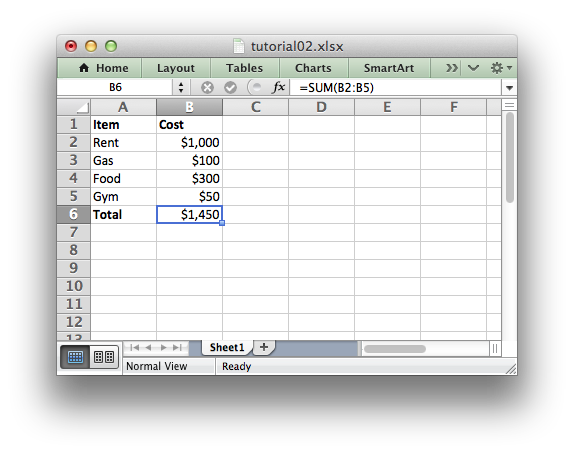libxlsxwriter
tutorial2.c

A tutorial program which is shown, with explanations, in Tutorial 2: Adding formatting to the XLSX File./*
* A simple program to write some data to an Excel file using the
* libxlsxwriter library.
*
* This program is shown, with explanations, in Tutorial 2 of the
* libxlsxwriter documentation.
*
* Copyright 2014-2021, John McNamara, jmcnamara@cpan.org
*
*/
#include "xlsxwriter.h"
/* Some data we want to write to the worksheet. */
struct expense {
char item;
int cost;
};
struct expense expenses[] = {
{"Rent", 1000},
{"Gas", 100},
{"Food", 300},
{"Gym", 50},
};
int main() {
/* Create a workbook and add a worksheet. */
lxw_workbook *workbook = workbook_new("tutorial02.xlsx");
lxw_worksheet *worksheet = workbook_add_worksheet(workbook, NULL);
int row = 0;
int col = 0;
int i;
/* Add a bold format to use to highlight cells. */
lxw_format *bold = workbook_add_format(workbook);
/* Add a number format for cells with money. */
lxw_format *money = workbook_add_format(workbook);
format_set_num_format(money, "\$#,##0");
/* Write some data header. */
worksheet_write_string(worksheet, row, col, "Item", bold);
worksheet_write_string(worksheet, row, col + 1, "Cost", bold);
/* Iterate over the data and write it out element by element. */
for (i = 0; i < 4; i++) {
/* Write from the first cell below the headers. */
row = i + 1;
worksheet_write_string(worksheet, row, col, expenses[i].item, NULL);
worksheet_write_number(worksheet, row, col + 1, expenses[i].cost, money);
}
/* Write a total using a formula. */
worksheet_write_string (worksheet, row + 1, col, "Total", bold);
worksheet_write_formula(worksheet, row + 1, col + 1, "=SUM(B2:B5)", money);
/* Save the workbook and free any allocated memory. */
return workbook_close(workbook);
}
workbook_close
lxw_error workbook_close(lxw_workbook *workbook)
Close the Workbook object and write the XLSX file.
worksheet_write_formula
lxw_error worksheet_write_formula(lxw_worksheet *worksheet, lxw_row_t row, lxw_col_t col, const char *formula, lxw_format *format)
Write a formula to a worksheet cell.
workbook_new
lxw_workbook * workbook_new(const char *filename)
Create a new workbook object.
format_set_bold
void format_set_bold(lxw_format *format)
Turn on bold for the format font.
format_set_num_format
void format_set_num_format(lxw_format *format, const char *num_format)
Set the number format for a cell.
lxw_worksheet
Struct to represent an Excel worksheet.
Definition: worksheet.h:2107
lxw_format
Struct to represent the formatting properties of an Excel format.
Definition: format.h:358
lxw_workbook
Struct to represent an Excel workbook.
Definition: workbook.h:280
worksheet_write_string
lxw_error worksheet_write_string(lxw_worksheet *worksheet, lxw_row_t row, lxw_col_t col, const char *string, lxw_format *format)
Write a string to a worksheet cell.
worksheet_write_number
lxw_error worksheet_write_number(lxw_worksheet *worksheet, lxw_row_t row, lxw_col_t col, double number, lxw_format *format)
Write a number to a worksheet cell.